Courses

# Notes | EduRev

## JEE : Notes | EduRev

The document Notes | EduRev is a part of the JEE Course Mock Test Series for JEE Main & Advanced 2021.
All you need of JEE at this link: JEE
• Current:- Current strength, in a conductor, is defined as the rate of flow of charge across any cross section of the conductor.
I= q/t = ne/t
For non-uniform flow,
I= dq/dt
Or, q = ? I dt
• Units of electric current:-
(a) C.G.S. electro-static unit (esu):- 1 esu of current (stat-ampere) = 1 esu of charge/1 second
(b) C.G.S. electro-static unit (emu):- 1 emu of current (ab-ampere) = 1 emu of charge/1 second
(c) S. I unit (ampere):- 1 ampere = 1 coulomb/1 second
(d) 1 A = 3×109esu of current or stat-ampere
(e) 1 A = 1/10 emu of current or abampere
• Drift velocity:-The velocity with which the free electrons are drifted towards the positive terminal, under the action of the applied field, is called the drift velocity of the free electrons.
V = (eV/ml)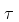Here, e is the charge of electron, V is the potential difference, m is the mass andis the relaxation time.
• Electric current and Drift velocity:-  I= q/t = nAve
• Ohm’s Law for conductors:- At constant temperature current flowing through a conductor of uniform area of cross-section, is proportional to the difference of potential across its terminals.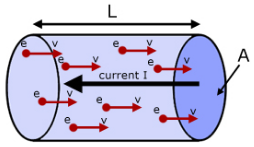(a) V = IR , Here, R = (ml/nAe2) (1/)

(b) R=ρl/A

(c) ρ = 1/σ

(d) vd = (qE/m)

(e) I = neAvd

(f) ρ = m/ne2(g) σ = ne2/m

• Resistance (R):- Resistance of a conductor is defined as the ratio between potential differences between the two ends of the conductor to the current flowing through it.
R= V/I
• Units of R:-
(a) In S.I:- 1 ohm = 1 volt/1 ampere
(b) In C.G.S system:-
1 statohm = 1 statvolt/1 statamp
1 abohm = 1 abvolt/1 abampere
(c) Relation between ohm and statohm:- 1 ohm = (1/9×1011) statohm
(d) Relation between ohm and abohm:- 1 ohm = 109abohm
• Variation of resistance with temperature:-
Temperature coefficient of resistance (α) is defined as change in resistance of the conductor per unit resistance per degree centigrade rise of temperature.
Rt=R0[1+α(T-T0)]
α = Rt - R0/R0(T-T0)
Here, Rt,R0 is the resistance of the conductor at tº C and 0º C respectively.
• Resistivity of material (ρ):- ρ = RA/l ,
Here R is the resisteance of the conductor, A is cross sectional area of conductor and l is the length of the conductor
• Relation between resistivity(ρ) and relaxation time (τ):-
ρ = m /ne2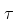• Variation of resistivity with temperature:-
(a) Conductors:-
ρt = ρ[1+α(T-T0)]
Here c is called the temperature coefficient of the resistivity.
= (ρt - ρ0) /ρ0 (T--T0)
Temperature coefficient of resistivity of a conductor is defined as the change in resistivity per unit resistivity per degree Celsius rise of temperature.
α=ρt - ρ0
(b) Insulators:-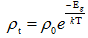• Conductivity (σ):- Conductivity of a material is defined as the reciprocal of the resistivity.
σ = 1/ρ
Unit:- ohm-1m-1
• Conductance:-Conductanceof a conductor is defined as the reciprocal of its resistance.
Conductance = 1/R = (1/ρ) (A/l)
Unit:- mho or ohm-1m-1
• Current Density:-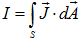(a) J = I/A
(b) J = nevd
(c) J =σE
(d) µ = vd/E
(e) σ = neµ
• Relation between current density and electrified:-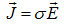Thus, electrical conductivity can also be defined as electric current density per unit electric field strength.
• Resistance in series:- If anumber of resistances are connected in series with each other, the net resistance of the combination is equal to the sum of their individual resistances.
(a) R = R1+R2+R3
(b) V = V1+V2+V3
(c) I = I1 = I= I3 = Constant
(d) V1 = IR1, V2 = IR2, V= IR3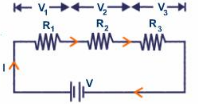• Resistance in parallel:- If a number of resistances are connected in parallel, the reciprocal of the resistance of the combination is equal to the sum of the reciprocals of their individual resistances.
(a) 1/R = 1/ R1 + 1/ R2 +1/ R3
(b) I = I1+I2+I3
(c) V = V1 = V= V3 = Constant
(d) I1 = V/R1, I2 = V/R2, I3 = V/R3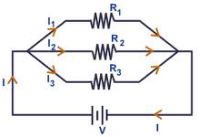• Distribution of current in a parallel combination of resistances:-
(a) I= I (R2/R1+R2)
(b) I2 = I (R1/R1+R2)
In general,
Current in one branch = total current × (resistance of second branch / sum of resistances in the two branches)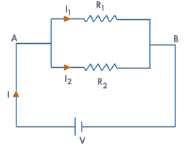• Grouping of cells:-
(a) Cells in series:-
I= (nE)/(R+nr)
If R<<nr, then I = E/R
If R>>nr, then I = nE/R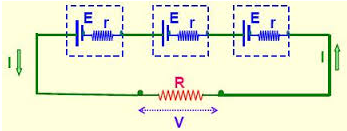(b) Cells in parallel:-
I= E/[R+(r/m)]
If R>>r/m, then I = E/R
If R>>r/m, then I = m(E/R)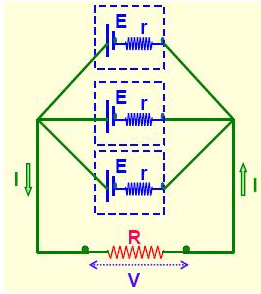(c) Mixed grouping:-
(a) I = mnE/(mR+nr)
(b) I is maximum when nR = mR
(c) Imax = mnE/(2√mnrR)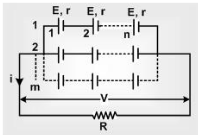• Electromotive force and potential difference:-
The electromotive force E of a cell is defined as the difference of potential between its terminals when there is no current in the external circuit, i.e., when the cell is in open circuit.
The potential difference of a cell is the difference of potential between two terminals when it is in closed circuit.
E = V+IR
• Internal resistance (r) of a cell:- The resistance offered by the electrolyte of the cell when the electric current passes through it is known as the internal resistance of the cell.
r = R (E-V/V)
• Electric Power:-
(a) P = VI
(b) P = I2R = V2/R
Unit of power:-1 watt = 1 volt × 1 amp
• Electric energy:-
W = Vq = V(It)
Unit of electric energy:-
1 joule = 1 watt sec
1 kilowatt hour = 1000 watt hour
• Faraday’s Laws of Electrolysis:-
(a) The mass of ion deposited on an electrode in the process of electrolysis, is proportional to the quantity of charge that has passed through the electrolyte.
m = Zq = ZIt
(b) When same current passes through several electrolytes for the same time, the masses of various ions deposited at each of the electrodes are proportional to their chemical equivalents (equivalent weights).
m/W = constant
Or, Z1/Z2 = W1/W2
So, W/Z = constant = F
• Heating effect of current:-
I = I2Rt  Joule = I2Rt/J Calorie
• Electric Bulb:-
(a) Resistance of filament, R = V2/P
(b) Maximum current that can be allowed to pass through bulb, Imax = P/V
• Total power consumed in parallel combination:-
P = P1+P2+P3
• Total power consumed in series combination:-
1/P= 1/P1+1/P2+1/P3
• Effect of stretching a resistance wire:-
R2/R1 = (l1/l2) (A1/A2) = (l2/l1)2 = (A1/A2)2 = (r1/r2)4      [Since, l1 A1 = l2 A2]
• Thermo e.m.f:-e = αθ + (βθ2/2)       (Here, θ = θH = θC)
• Neutral temperature: θN = -(α/β)
• Temperature of inversion:- θN = (θ+ θC)/2 [Since, θ1 – θ= θN – θC]
• Thermoelectric power or Seebeck Coefficient:- S =de/dθ = α +βθ
• Peltier effect:-
Heat absorbed per second at a junction when a current I flows = πI
Here π is Peltier coefficient and is given by, π = SθH
• Thomson coefficient:-
σ = (ΔQ/time)/IΔθ
Offer running on EduRev: Apply code STAYHOME200 to get INR 200 off on our premium plan EduRev Infinity!

## Mock Test Series for JEE Main & Advanced 2021

6 videos|264 docs|178 tests

,

,

,

,

,

,

,

,

,

,

,

,

,

,

,

,

,

,

,

,

,

;